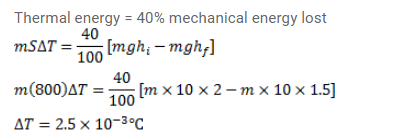# A ball is droppedon a floor from a height of 2.0 m.Question:

A ball is droppedon a floor from a height of $2.0 \mathrm{~m}$. After the collision it rises up to a height of $1.5 \mathrm{~m}$. Assume that $40 \%$ of the mechanical energy lost goes as thermal energy into the ball. Calculate the rise in the temperature of the ball in the collision. Heat capacity of the ball is $800 \mathrm{~J} / \mathrm{K}$.

Solution: